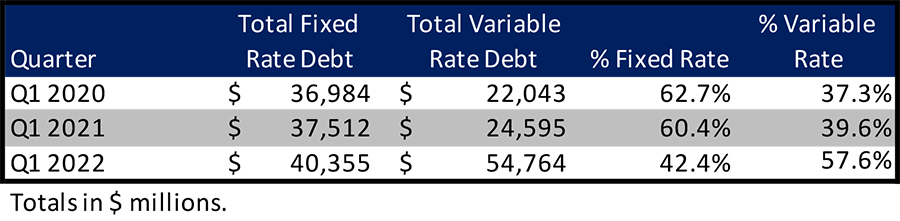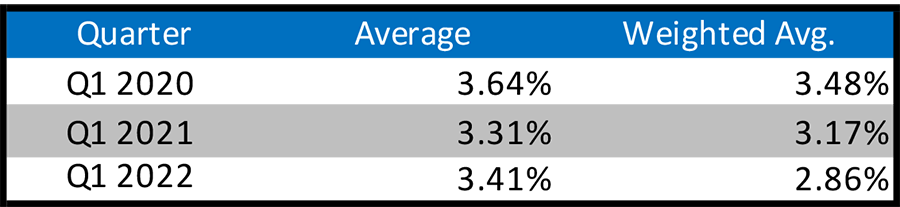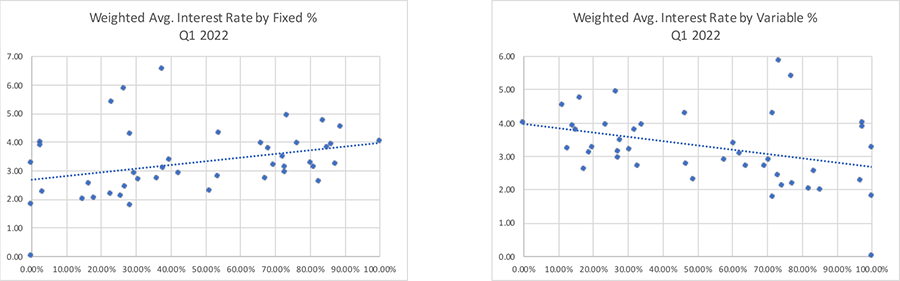July 8, 2022

# Nontraded REITs Use More Variable Rate Debt with Wider Dispersion in Interest RatesJuly 8, 2022

# Nontraded REITs Use More Variable Rate Debt with Wider Dispersion in Interest Rates

July 8, 2022 | James Sprow | Blue Vault

As the era of historically low interest rates draws to a close, we can see how the increased use of variable rate debt by nontraded REITs over the last two years has both lowered the weighted average interest rates being paid by those REITs and increased the dispersion of rates across the NTRs. As noted in a previous article, the proportion of variable rate debt on NTR balance sheets has increased dramatically since Q1 2020, going from 37.3% of all debt to 57.6% in just two years. Blackstone REIT’s large influence on both the use of variable rate debt and the proportion of variable rate debt is shown in the REIT’s increase from 38.9% variable rate debt in Q1 2020 to 57.6% variable rate debt in Q1 2022. The REIT’s use of variable rate debt almost perfectly mirrors the proportion used by all NTRs.With the increased use of variable rate debt, the weighted average interest rate on all NTR debt has fallen from 3.48% in Q1 2020 to 2.86% Q1 2022. As the indices that determine the rates on variable rate debt increase this year, we can expect more of the nontraded REITs to have upward adjustments in the weighted average interest rates on their debt, both due to the higher index rates and their greater use of variable rate debt.

The charts below show the relationship between the proportion of debt at variable (effectively unhedged) rates and the weighted average interest rates for the active nontraded REITs as of March 31, 2020, and March 31, 2022. Note the negative (favorable) relationship between the proportion of debt at variable rates and the weighted average interest rates reported by the REITs.These two charts illustrate that the low variable rates that pertained as of March 31, 2020, have led REITs to use more variable rate debt, and the result has been a wider dispersion in the effective weighted average interest rates on their total debt.

As of July 7, 2022, the 30-day LIBOR rate stood at 1.87%, up from 0.12% as of March 31, 2020. The dual effect on borrowing costs for nontraded REITs resulting from the larger proportion of debt at variable rates and the lower rates on that debt tied to the historically low LIBOR rate will now reverse. As LIBOR and the newer index of SOFR increase with the general trend for interest rates in the U.S. and globally, nontraded REITs with large portions of their borrowings at variable rates will see substantial increases in interest expense. Also, in volatile interest rate environments that have not been the norm for several years, the cost of swapping variable rates for fixed rates via swap contracts will increase. Interest rate caps that are in place should become “binding” as rates reach the cap rates, but not all variable rate debt has caps in place.

Blackstone REIT, the largest nontraded REIT with the largest amount of variable rate debt as of March 31, 2022, had a total notional amount of interest rate caps of \$14.4 billion as of that date. The REIT also had interest rate swaps in place on their property debt with a notional amount of \$15.1 billion. The REIT had 31 interest rate swaps and 33 interest rate caps in place as of the end of Q1 2022. From the REIT’s Q1 2022 10-Q, “The Company uses derivative financial instruments, which includes interest rate swaps, and may also include interest rate caps, options, floors, and other interest rate derivative contracts, to limit the Company’s exposure to the future variability of interest rates.” The fair value of these derivative contracts was \$826 million as of the end of the quarter, up from just \$41 million as of December 31, 2021. This increase in fair value reflects the impact of rising rates on the value of the hedging contracts in place.

Conclusions

Nontraded REITs have increasingly used variable rate financing to take advantage of the low interest rate environment since Q1 2020. Along with the lower weighted average interest rates that the REITs were paying on their debt, the trend toward use of variable rate debt has led to increased vulnerability to higher rates going forward. We see evidence of this vulnerability in the greater dispersion of average interest rates across the REITs since Q1 2020. While caps and swaps can protect nontraded REITs from higher borrowing rates on variable rate contracts, we can expect those hedging instruments to become more expensive and weighted average interest rates to increase for many nontraded REITs.

Sources:  Blue Vault, SEC